# Excercise 3.1 Understanding Quadrilaterals- NCERT Solutions Class 8

## Chapter 3 Ex.3.1 Question 1

Given here are some figures.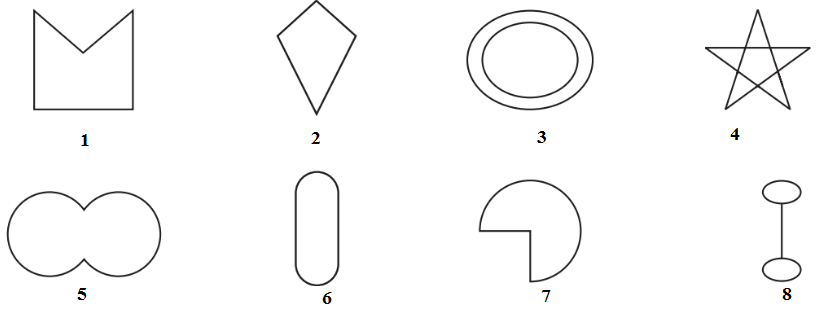Classify each of them on the basis of the following.

(a) Simple curve

(b) Simple closed curve

(c) Polygon

(d) Convex polygon

(e) Concave polygon

### Solution

Steps:

(a) Simple curve - A simple curve is a curve that does not cross itself.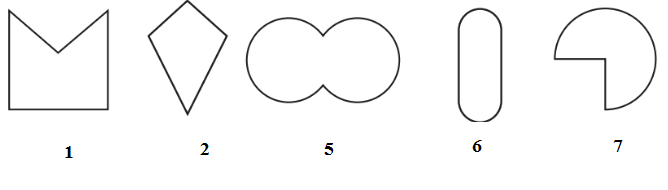(b) Simple closed curve -In simple closed curves the shapes are closed by line-segments or by a curved line.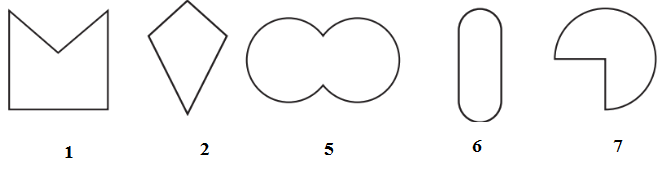(c) Polygon-A simple closed curve made up of only line segments is called a polygon.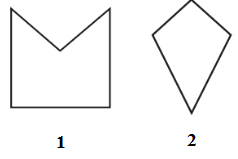(d) Convex polygon-A Convex polygon is defined as a polygon with no portions of their diagonals in their exteriors.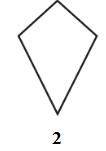(e) Concave polygon- A concave polygon is defined as a polygon with one or more interior angles greater than $$180^{\circ}.$$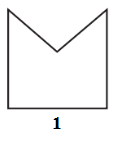## Chapter 3 Ex.3.1 Question 2

How many diagonals does each of the following have?

(b) A regular hexagon

(c) A triangle

### Solution

What is known?

Polygons with known number of vertices.

What is unknown?

Number of diagonals

Reasoning:

A diagonal is a line segment connecting two non-consecutive vertices of a polygon. Draw the above given polygon and mark vertices and then, draw lines joining the two non-consecutive vertices. From this, we can calculate the number of diagonals.

A convex quadrilateral has two diagonals.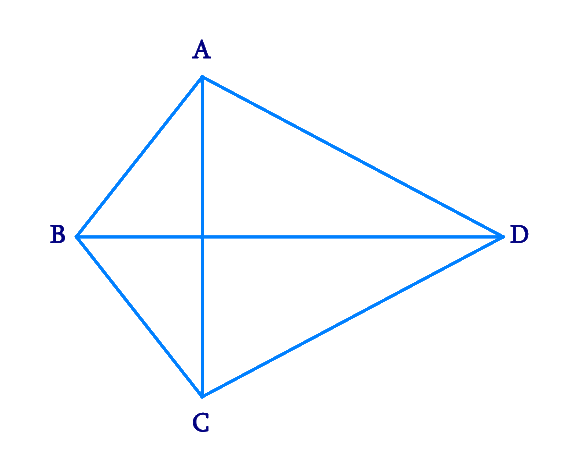Here, $$AC$$ and $$BD$$ are two diagonals.

(b) A regular hexagon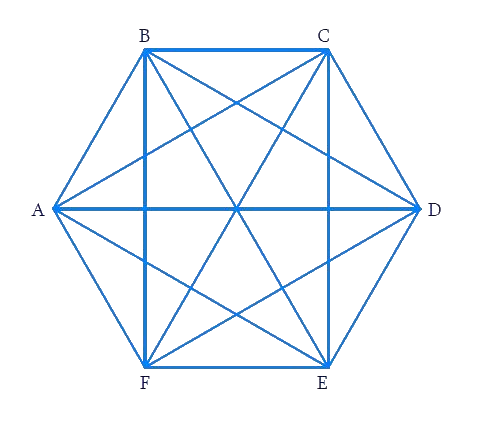Here, the diagonals are $$AD, \,AE,\, BD,\, BE,\, FC,\, FB, \,AC,\, EC$$ and $$FD.$$ Totally there are $$9$$ diagonals.

(c) A triangle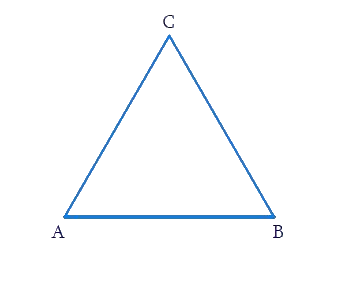A triangle has no diagonal because there no two non-consecutive vertices.

## Chapter 3 Ex.3.1 Question 3

What is the sum of the measures of the angles of a convex quadrilateral? Will this property hold if the quadrilateral is not convex? (Make a non-convex quadrilateral and try!)

What is known?

Quadrilateral $$ABCD$$

What is unknown?

Sum of the measures of the angles of a convex quadrilateral.

Reasoning:

Let $$ABCD$$ be a convex quadrilateral. Then, we draw a diagonal $$AC$$ which divides the Quadrilateral into two triangles. We know that the sum of the angles of a triangle is $$180$$ degree, so by calculating the sum of the angles of a $$∆ABC$$ and , we can measure the sum of angles of convex quadrilateral.

### Solution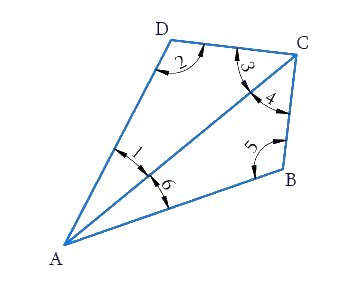$$ABCD$$ is a convex quadrilateral made of two triangles $$∆ABC$$ and $$∆ADC.$$ We know that the sum of the angles of a triangle is $$180$$ degree. So:

\begin{align}\!\angle 6\! +\! \angle 5 \!+ \!\angle 4\! =\! {180^ \circ }\left[ \begin{array}{l}{\rm{Sum}}\,\,{\rm{of}}\,{\rm{the}}\,{\rm{angles}}\,\\{\rm{of}}\,{\rm{\Delta ABC}}\,{\rm{ = 18}}{{\rm{0}}^{\rm{o}}}\end{array} \right]\\ \angle 1\!+ \!\angle2\!+\!\angle 3\!=\!{180^ \circ }\left[ \begin{array}{l}{\rm{Sum}}\,\,{\rm{of}}\,{\rm{the}}\,{\rm{angles}}\,\\{\rm{of}}\,{\rm{\Delta ADC}}\,{\rm{ = 18}}{{\rm{0}}^{\rm{o}}}\end{array} \right] \end{align}

\begin{align}\angle 6 &+ \angle 5 + \angle 4 + \angle 1 + \angle 2 + \angle 3\\ &= {{180}^{\rm{o}}} + {{180}^{\rm{\circ}}}\\ & = {{360}^{\rm{\circ}}}\end{align}

Hence, the sum of measures of the triangles of a convex quadrilateral is $$360^\circ$$. Yes, even if quadrilateral is not convex then, this property applies. Let $$ABCD$$ be a non-convex quadrilateral; join $$BD$$, which also divides the quadrilateral in two triangles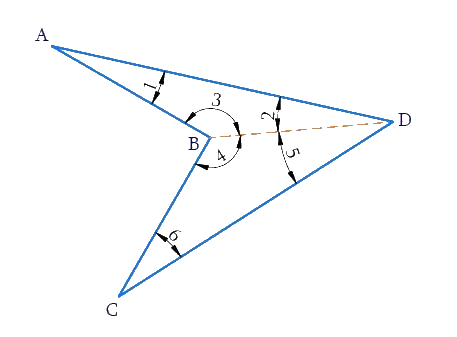Using the angle sum property of triangle, again$$ABCD$$ is a concave quadrilateral, made of two triangles $$\Delta \text{ABD}$$ and $$\Delta \text{BCD}$$ .Therefore, the sum of all the interior angles of this quadrilateral will also be,

$180^\circ + 180^\circ=360^\circ$

## Chapter 3 Ex.3.1 Question 4

Examine the table. (Each figure is divided into triangles and the sum of the angles deduced from that.)

 FIGURES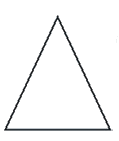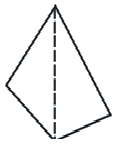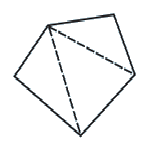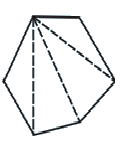SIDE 3 4 5 6 ANGLE SUM $$180$$ \begin{align} 2 \times {180^{\rm{o}}} & = (4 - 2) \times {180^{\rm{o}}}\\ &= 360^\circ \end{align} \begin{align} 3 \times {180^{\rm{o}}}\\ & = (5 - 2) \times {180^{\rm{o}}}\\ & = 540 \end{align} \begin{align} 4 \times {180^{\rm{o}}}\\ & = (6 - 2) \times {180^{\rm{o}}}\\ & = {720^{\rm{o}}} \end{align}

What can you say about the angle sum of a convex polygon with number of sides?

(a) $$7$$

(b) $$8$$

(c) $$10$$

(d) $$n$$

### Solution

From the table, it can be observed that the angle sum of a convex polygon of $$n$$ sides is $$\left( {n - 2} \right){\rm{ }} \times {\rm{ }}180^\circ$$

(a) When $$n = 7$$

Then Angle sum of a polygon

\begin{align} &= (n - 2) \times {180^{\rm{\circ}}} \\&= (7 - 2) \times {180^{\rm{\circ}}} \\&= 5 \times {180^{\rm{\circ}}} \\&= {900^{\rm{\circ}}}\end{align}

(b) When $$n = 8$$

Then Angle sum of a polygon

\begin{align} &= (n - 2) \times {180^{ \rm{ \circ}}} \\&= (8 - 2) \times {180^{\rm{\circ}}} \\&= 6 \times{180^{\rm{\circ}}} \\&= {1080^{\rm{\circ}}}\end{align}

(c) When $$n = 10$$

Then Angle sum of a polygon

\begin{align} &= (n - 2) \times {180^{\rm{\circ}}} \\&= (10 - 2) \times {180^{\rm{\circ}}} \\&= 8 \times {180^{\rm{\circ}}} \\&= {1440^{\rm{\circ}}}\end{align}

(d) When $$n = n$$

Then Angle sum of a polygon $$= \left( {n - {\rm{ }}2} \right) \times 180^\circ$$

## Chapter 3 Ex.3.1 Question 5

What is a regular polygon? State the name of a regular polygon of

(i) $$3$$ sides

(ii) $$4$$ sides

(iii) $$6$$ sides

### Solution

What is known?

Number of sides of polygon

What is unknown?

Name of a regular polygon of

(i) $$3$$ sides

(ii) $$4$$ sides

(iii) $$6$$ sides

Steps:

Regular polygon - A polygon having all sides of equal length and the interior angles of equal measure is known as regular polygon i.e. a regular polygon is both ‘equiangular’ and ‘equilateral

(i) $$3$$ sides $$=$$ polygons having three sides is called a Triangle.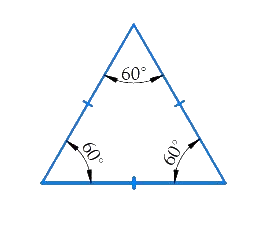(ii) $$4$$ sides $$=$$ polygons having four sides is called square/quadrilateral.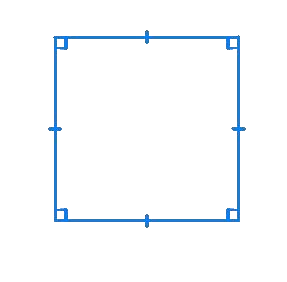(iii) $$6$$ sides $$=$$ polygons having six sides is called a Hexagon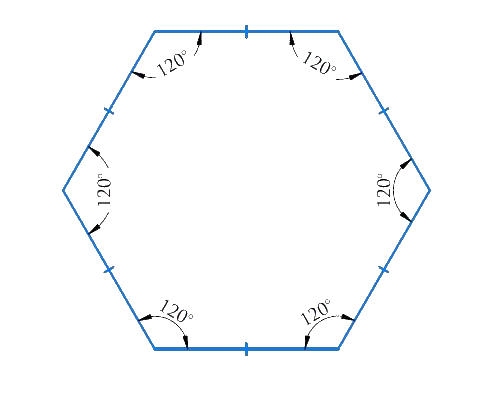## Chapter 3 Ex.3.1 Question 6

Find the angle measure $$x$$ in the following figures: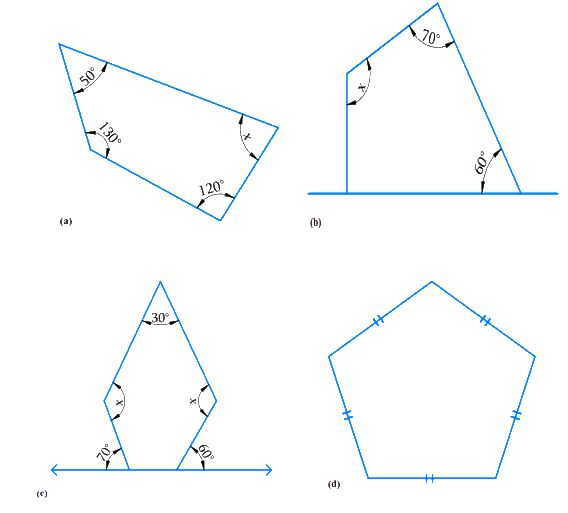### Solution

What is Known?

Sum of the measures of all interior angles of a quadrilateral is $$360^\circ$$ and that of a pentagon is $$540^\circ$$

What is Unknown?

Angle $$x$$ in the above figures  $$a, b, c$$ and $$d$$

Steps: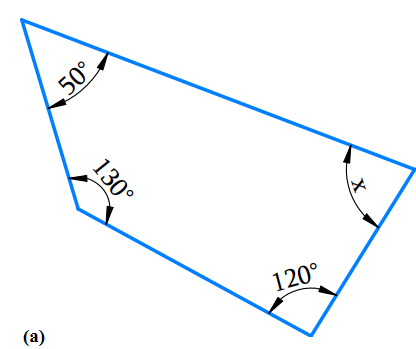a) The above figure has $$4$$ sides and hence it is a quadrilateral.

Using the angle sum property of a quadrilateral,

\begin{align}{{50}^{\rm{o}}} + {{130}^{\rm{o}}} + {{120}^{\rm{o}}} + x &= {{360}^{\rm{o}}}\\ {{300}^{\rm{o}}} + x &= {{360}^{\rm{o}}}\\x & = {{360}^{\rm{o}}} - {{300}^{\rm{o}}}\\x &= {{60}^{\rm{o}}} \end{align}

b) Using the angle sum property of a quadrilateral.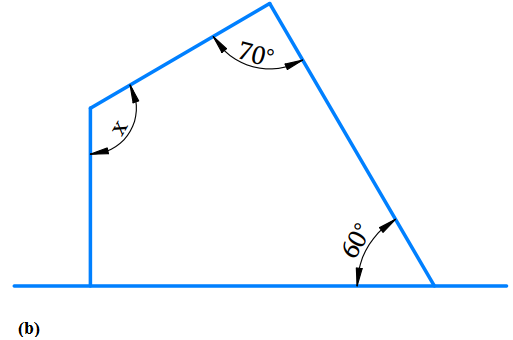\begin{align}{{90}^\circ } + {{60}^\circ } + {{70}^\circ } + x& = {{360}^\circ }\\{{220}^\circ } + x &= {{360}^\circ }\\{220^\circ } + x &= {360^\circ }\\x& = {360^\circ } - {220^\circ }\\x &= {140^\circ }\end{align}

c) The given figure is a pentagon $$(n=5)$$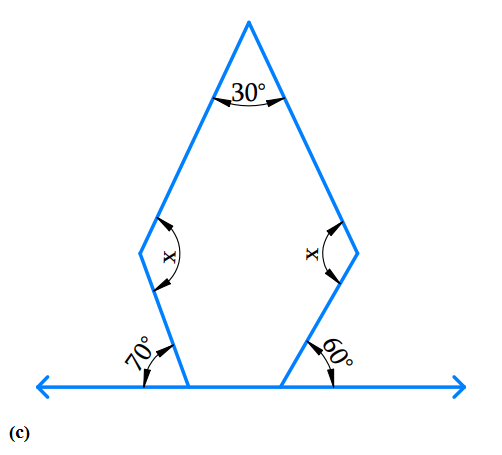Angle sum of a polygon

\begin{align}& = ({\rm{n} - 2) \times {{180}^{\rm{o}}}}\\&=(5 - 2) \times {{180}^{\rm{o}}}\\& =3 \times {{180}^{\rm{o}}}\\ & = {540}^{\rm{o}}\end{align}

Sum of the interior angle of pentagon is $$540^\circ$$

Angles at the bottom are linear pair.

$$\therefore$$ First base interior angle a

\begin{align}& = {180^{\rm{o}}} - {70^{\rm{o}}}{\rm{ }}\left( \begin{array}{l}{\text{angle of straight }}\\{\text{line is }}{180^{\rm{o}}}\end{array} \right)\\ &= {\mkern 1mu} {\mkern 1mu} {110^{\rm{o}}} \end{align}

$$\therefore$$  Second base interior angle b

\begin{align}&= {{180}^{\rm{o}} - {{60}^{\rm{o}}}}\\& = {{120}^{\rm{o}}}\end{align}

Angle sum of a polygon $$= ({\rm{n}} - 2) \times {180^o}$$

\begin{align}\\{30^o} + x + {110^o} + {120^o} + x &= {540^o}\\2x + {260^{\rm{o}}}\, &= {540^{\rm{o}}}\\2x &\!=\!{540^{\rm{o}}}\!-\!{260^{\rm{o}}}\\2x &= {280^{\rm{o}}}\\x &= \frac{{{{280}^{\rm{o}}}}}{2}\\x &= {140^{\rm{o}}}\end{align}

D) The given figure is pentagon $$(n-5)$$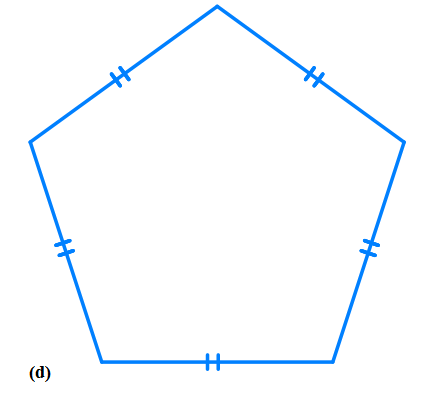Angle sum of a polygon

\begin{align}& = ({\rm{n}} - 2) \times {{180}^{\rm{o}}}\\& = (5 - 2) \times {{180}^{\rm{o}}}\\& = 3 \times {{180}^{\rm{o}}}\\& = {{540}^{\rm{o}}}\end{align}

Sum of the interior angle of pentagon is $$540^\circ$$

Angle sum of a polygon

\begin{align}&= {x + x + x + x + x = {{540}^{\rm{o}}}}\\{\rm{ i}}{\rm{.e}}{\rm{. }}\ \qquad 5x &= {{540}^{\rm{o}}}\\x &= {\frac{{{{540}^{\rm{o}}}}}{5}}\\x & = {{108}^{\rm{o}}}\end{align}

A pentagon is a regular polygon i.e. it is both ‘equilateral’ and ‘equiangular’. Thus, the measure of each interior angle of the pentagon are equal.

Hence each interior angle is $$108^\circ .$$

## Chapter 3 Ex.3.1 Question 7

a) Find $$x +y +z$$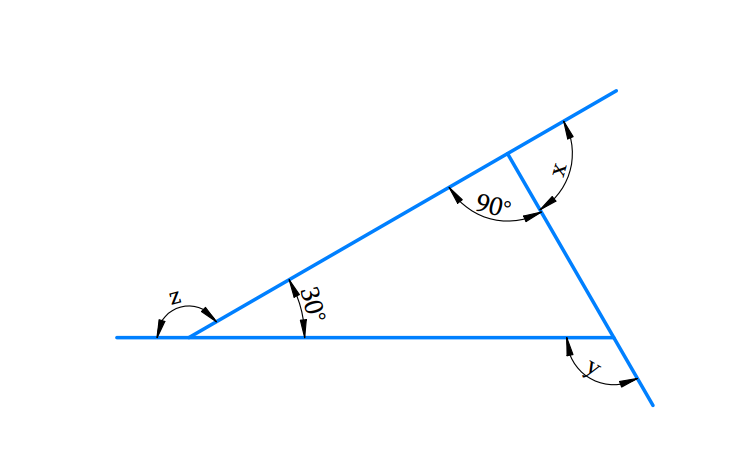b) Find $$x +y +z +w$$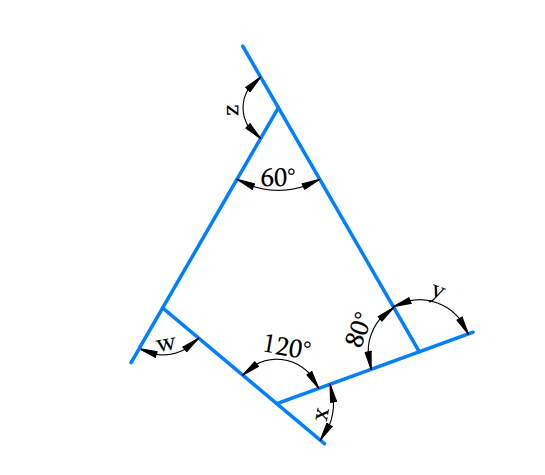### Solution

What is Known?

The sum of the measures of all the interior angles of a quadrilateral is $$360^\circ$$ and that of a triangle is $$180^\circ$$

What is Unknown?

Angle$$x, y, z$$ and $$w$$ in the above figures $$a$$ and $$b$$

Reasoning:

The unknown angles can be estimated by using the angle sum property of a quadrilateral and triangle accordingly.

Steps:

(a) Find $$x +y +z$$Sum of linear pair of angles is$$= {180^{\rm{o}}}$$

\begin{align}x + {90^{\rm{o}}} & = {180^{\rm{o}}}{\text{ (Linear pair)}}\\x & = {180^{\rm{o}}} - {90^{\rm{o}}}\\x & = {90^{\rm{o}}}\end{align}

And

\begin{align}z + 30^{\rm{o}} &= {180^{\rm{o}}}{\rm{ }}({\text{Linear pair}})\\z & = 180^{\rm{o}} - {30^{\rm{o}}}\\z &= {150^{\rm{o}}}\end{align}

And

\begin{align}y & ={{90}^{\text{o}}}+{{30}^{\text{o}}}\text{ }\left( \begin{array}&\text{Exterior angle } \\ \text{theorem} \\ \end{array} \right) \\ y & ={{120}^{\text{o}}} \\\end{align}

\begin{align}x + y + z & = {90^{\rm{o}}} + {120^{\rm{o}}} + {150^{\rm{o}}}\\& = {360^{\rm{o}}}\end{align}

(b)Find $$x +y +z$$The sum of the measures of all the interior angles of a quadrilateral is $$360^\circ.$$

Using the angle sum property of a quadrilateral,

Let $$n$$ is the fourth interior angle of the quadrilateral.

\begin{align}6{{0}^{\text{o}}}+8{{0}^{o}}+12{{0}^{o}}+n &=36{{0}^{o}} \\26{{0}^{o}}+n& =36{{0}^{o}} \\n& =36{{0}^{o}}-26{{0}^{o}} \\n& =10{{0}^{o}} \\\end{align}

sum of linear pair of angles is $$= {180^{\rm{o}}}$$

\begin{align}w + 100^\circ & = 180^\circ {\rm{ }} & \ldots \left( 1 \right)\\x + 120^\circ & = 180^\circ & \ldots \left( 2 \right)\\y + 80^\circ & = 180^\circ & \ldots \left( 3 \right)\\z + 60^\circ & = 180^\circ & \ldots \left( 4 \right)\end{align}

Adding equation ($$1), (2), (3)$$and $$(4),$$

\begin{align} & \left( \begin{array} & w\!+\!{{100}^{{}^\circ }}\!+\!x+{{120}^{{}^\circ }} \\ \!+\!y\!+\!{{80}^{{}^\circ }}\!+\!z+{{60}^{{}^\circ }} \\ \end{array} \right)\!\!=\!\!\left( \begin{array} & {{180}^{{}^\circ }}\!+\!{{180}^{{}^\circ }}\!+\! \\ {{180}^{{}^\circ }}\!+\!{{180}^{{}^\circ }} \\ \end{array} \right) \\&\,\, \quad w+x+y+z+{{360}^{{}^\circ }}={{720}^{{}^\circ }} \\ &\,\,\qquad \qquad w+x+y+z={{720}^{{}^\circ }}-{{360}^{{}^\circ }} \\ & \qquad \quad\,\,\; w+x+y+z={{360}^{{}^\circ }} \end{align}

The sum of the measures of the external angles of any polygon is $$360^\circ$$ .

Related Sections
Related Sections
Instant doubt clearing with Cuemath Advanced Math Program